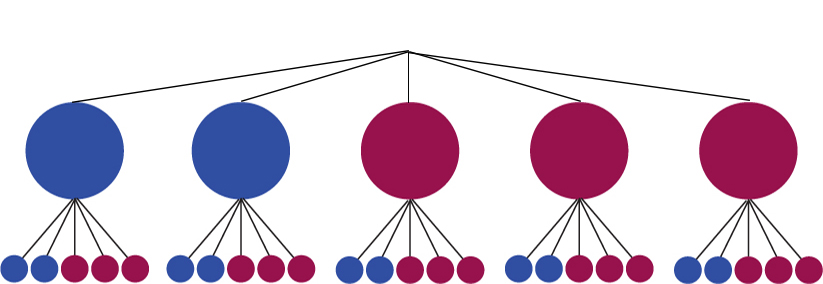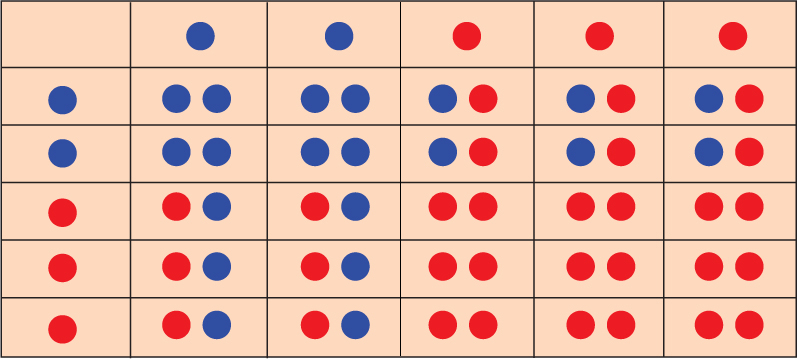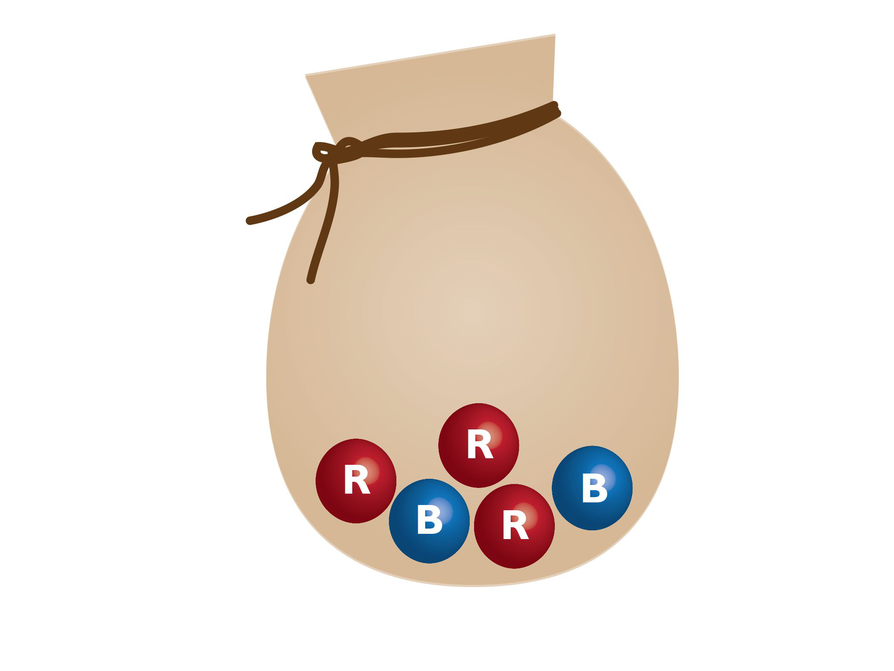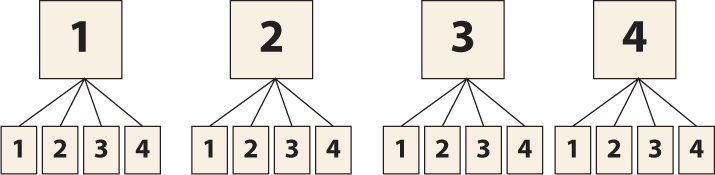Subject:
Statistics and Probability
Material Type:
Lesson Plan
Level:
Middle School
7
Provider:
Pearson
Tags:
• Causality
• Independent and Dependent Events
• Probability
Language:
English
Media Formats:
Text/HTML

# Fundamental Counting Principle## Overview

Students will continue to apply their understanding of compound independent events. They will calculate probabilities and represent sample spaces with visual representations.

# Key Concepts

• Students continue to solve problems with compound events. The formula for calculating the probability of independent events is introduced:
P(A and B) = P(A) ⋅ P(B)

# Goals and Learning Objectives

• Deepen understanding of compound events using lists, tables, and tree diagrams.
• Learn about the Fundamental Counting Principle.

# Lesson Guide

Have students read the Opening question. Give students a few minutes to think about the question and then have them share their thoughts.

ELL: Be sure that your pace is appropriate when posing this question, especially when interacting with ELLs.

SWD: If students are not observing why there are 72 combinations for shirts and pants, thinking aloud is one strategy for making learning visible. When teachers think aloud, they are externalizing their internal thought processes. Doing so may provide students with insights into mathematical thinking and ways of tackling problems. It also helps to model accurate mathematical language.

# Mathematics

Discuss student responses. Students will likely see that each of the 9 shirts can be paired with each of the 8 pants, making 72 combinations. Summarize the results from the previous lesson:

• Two coins: 2 outcomes for the first event, 2 for the second, 4 total outcomes
• One number cube and one coin: 6 outcomes for the first event, 2 for the second event, 12 total outcomes
• Two number cubes: 6 outcomes for the first event, 6 for the second, 36 total outcomes

Elicit from students that the number of outcomes for each event can be multiplied to find the total number of outcomes. Students may begin to see that the probability of each outcome can also be multiplied to find the probability of the compound event, although this idea is left for students to bring up and/or discover.

# Mathematical Practices

Mathematical Practice 7: Look for and make use of structure.

• The Opening allows students to see the relationship between sample space diagrams and the total number of outcomes for compound events.

# How Many Outfits?

Bob has nine different shirts and eight different pairs of pants.

• How many different outfits can he wear (using one shirt and one pair of pants)?# Lesson Guide

Discuss the Math Mission. Students will find the probability of compound events.

## Opening

Find the probability of compound events.

# Lesson Guide

Students can work individually or with a partner.

# Interventions

Student struggles to get started on the marble problem.

• How many outcomes are there for each event?
• If a marble is drawn, what are the outcomes for the second event?

Student has a solution but does not provide an explanation.

• Why did you approach the problem the way you did?
• Explain your strategy for solving the problem.

SWD: Struggling students may still need explicit instruction and guided scaffolding to recognize the relationships creating ratios to represent the probability and for multiplying fractions to find probability. Provide small group instruction to make sure that they can multiply fractions to find the probability accurately, and to convert fractions to decimals to percents.

• Answers will vary. Students will present a tree diagram, a list, or a table. Tree diagram:List: B, B| B, B| B, R| B, R| B, R
B, B| B, B| B, R| B, R| B, R
R, B| R, B| R, R| R, R| R, R
R, B| R, B| R, R| R, R| R, R
R, B| R, B| R, R| R, R| R, R

Table:• P(R then B) = $\frac{6}{25}$ = 0.24 = 24%

# Marbles

You have a bag of three red marbles and two blue marbles. You draw one marble, replace it, and draw a second marble.

• Represent the sample space with a tree diagram, a list, or a table.
• What is the probability that you will draw a red marble and then draw a blue marble?## Hint:

• How many marbles does the bag contain in all?
• What is the probability of selecting one red marble?
• Can you show the sample space in a tree diagram?

# Lesson Guide

Students can work individually or with a partner.

# Interventions

Student has an incorrect solution.

• How many outcomes are there for each event?
• Did you pair each of the first outcomes with each of the second outcomes?
• How many outcomes are favorable?

Student has a solution but does not provide an explanation.

• How did you know that multiplying would work?

• P(Karen selects a 2 the first time) = $\frac{1}{4}$ = 0.25 = 25%
• P(Karen selects a 2 the second time) = $\frac{1}{4}$ = 0.25 = 25%
• Tree diagram:Table:| 1| 2| 3| 4
---|---|---|---|---
1| 1, 1| 1, 2| 1, 3| 1, 4
2| 2, 1| 2, 2| 2, 3| 2, 4
3| 3, 1| 3, 2| 3, 3| 3, 4
4| 4, 1| 4, 2| 4, 3| 4, 4
List:
• 1, 1
• 1, 2
• 1, 3
• 1, 4
• 2, 1
• 2, 2
• 2, 3
• 2, 4
• 3, 1
• 3, 2
• 3, 3
• 3, 4
• 4, 1
• 4, 2
• 4, 3
• 4, 4
• P(Karen will select 2 both times) = $\frac{1}{16}$ = 0.0625 = 6.25%

# Cards

Karen has four cards numbered 1, 2, 3, and 4. She shuffles the cards face down, selects one randomly, and notes the number. Then she replaces the card, shuffles again, and selects a second card.

• What is the probability that Karen will select a 2 the first time she chooses a card?
• What is the probability that Karen will select a 2 the second time?
• Illustrate the sample space with a tree diagram, a list, or a table.
• What is the probability that Karen will select a 2 both times?

## Hint:

• How many cards does Karen have in all?
• If she selects a 2, how would she determine the probability of the next card she chooses?
• How can you use your representation of the sample space to help you solve the problem?

# Mathematics

As students work, look for students who do not draw the sample space or use an abbreviated version. Some students will see the number of outcomes without the sample space, but will realize that they might still need the sample space to count all of the favorable outcomes. Some students will take the next step, thinking of ways they can find the favorable outcomes without drawing the sample space.

# Mathematical Practices

Mathematical Practice 1: Make sense of problems and persevere in solving them.

• Students continue to build their understanding and fluency by calculating the theoretical probability of events.

Mathematical Practice 2: Reason abstractly and quantitatively.

• The Challenge Problem provides students with the symbolic representation of the probability of compound independent events, and has them solve Work Time problems using it rather than more concrete representations.
• Presentations will vary. Presentations should compare methods and explain when and why one type might be better than another.

# Challenge Problem

Marbles: P(draw red, then blue) = $\frac{3}{5}$$\frac{2}{5}$ = $\frac{6}{25}$

Cards: P(Karen draws a 2, then draws 2) = $\frac{1}{4}$$\frac{1}{4}$ = $\frac{1}{16}$

# Prepare a Presentation

Compare the methods of using tree diagrams, tables, and organized lists to find the probability of compound independent events.

# Challenge Problem

Karen says that compound probability of two independent events both occurring, then the probability is:

P(A and B) = P(A) • P(B)

• Use the formula to calculate the probability you will draw a red marble and then draw a blue marble.
• Use the formula to calculate the probability that Karen will select a 2 both times.

# Lesson Guide

Have student present their observations about different methods for representing sample spaces.

# Mathematics

• How did you find the sample space and the number of favorable outcomes?
• How did you determine the probability?

ELL: Call on ELLs and encourage them to participate, even if their pace is slower or they are reluctant to volunteer due to their weaker command of the language.

# Ways of Thinking: Make Connections

Take notes about the approaches your classmates used to find the probability of compound independent events.

## Hint:

• How does the diagram of the sample space help you solve the problem?
• Where do you see the probability in the diagram?
• Can you explain how you used the formula for finding the probability of a single event to find the probability of compound events?

# A Possible Summary

A compound event is two or more events that happen at the same time or one right after the other. Finding the probability is still the same: the ratio of the number of favorable outcomes to the total number of outcomes. Tree diagrams, systematic lists, and tables are tools used to find the sample space, which is a list of all possible outcomes. If the events of a compound event are independent, you can also find the probability of a compound event by multiplying together the probability of each event.

ELL: ELLs will understand more of what you say if you remember to modify your language as needed. Find subtle but effective ways to check that students have understood.

• Use shorter, less complex sentences for beginning ELLs.
• Avoid pronouns.
• Emphasize nouns and verbs.
• Use simple sentence structures.
• Use intonation, volume, and pauses to help make the meaning clear.
• Paraphrase important concepts and directions.

# Summary of the Math: Compound Events

Write a summary about finding the probability of compound events using diagrams and a formula.

## Hint:

• Do you explain how to determine the probability of compound independent events?